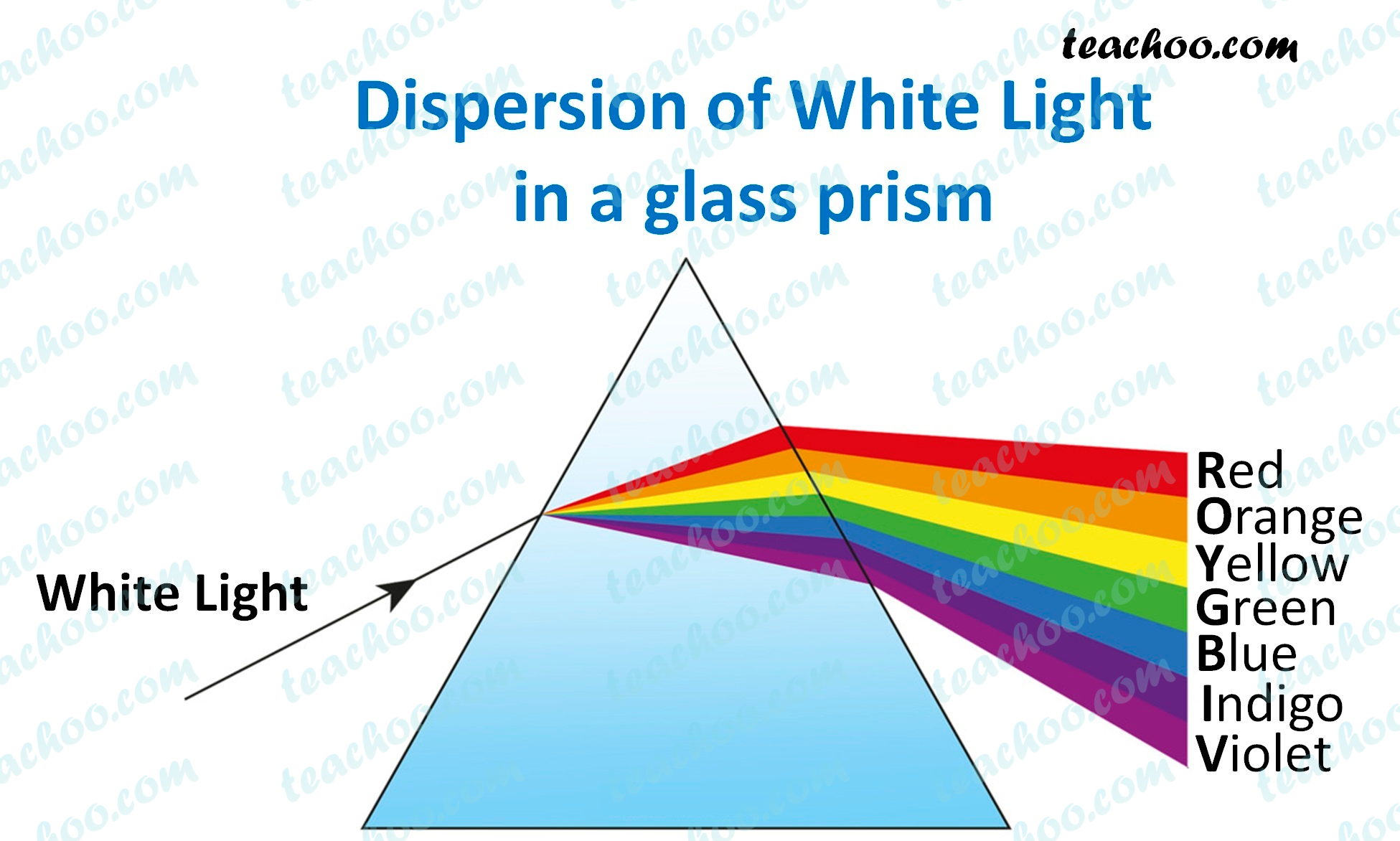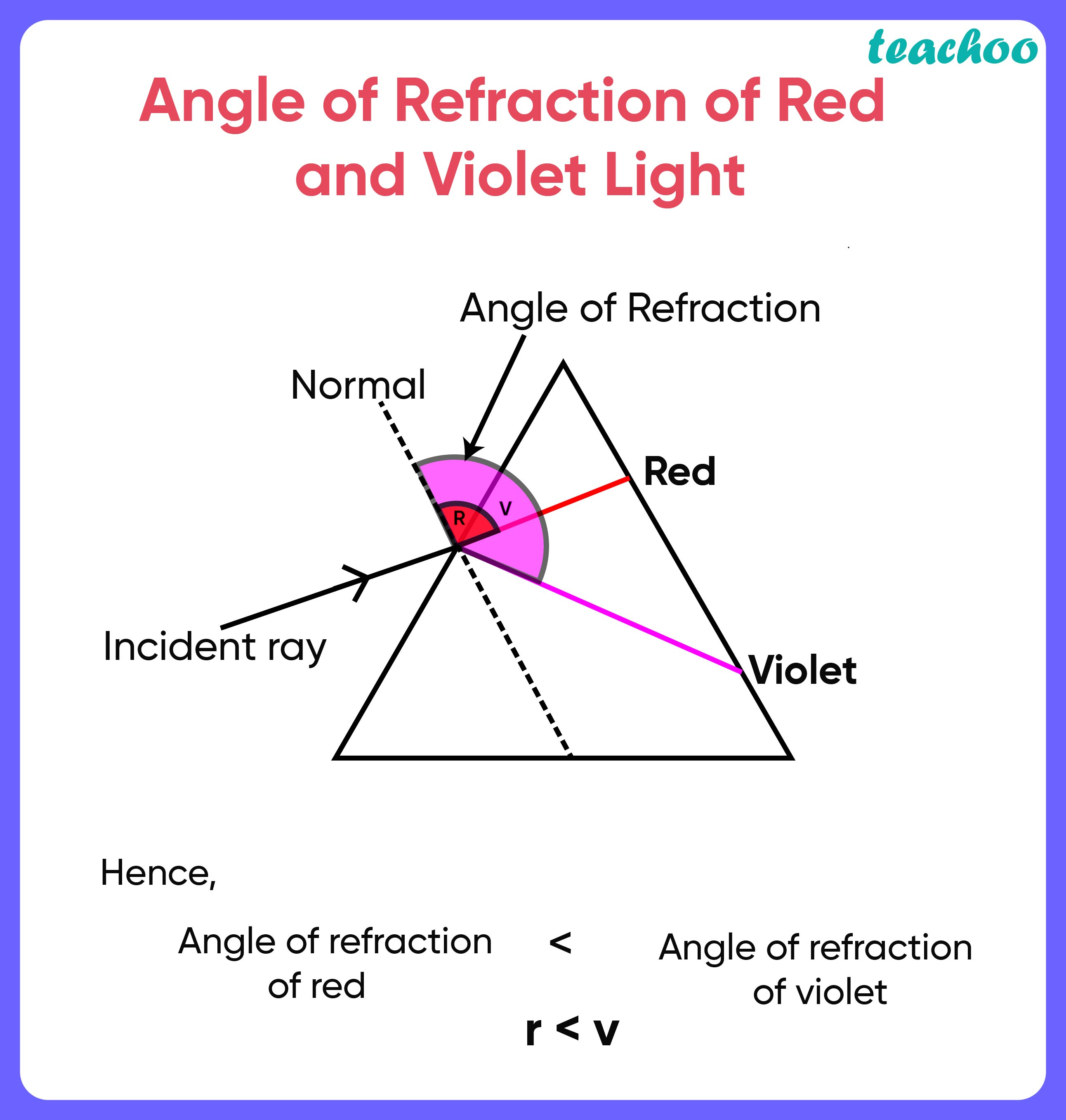Solutions - CBSE Class 10 Sample Paper for 2022 Boards - Science [MCQ]

Class 10
Solutions to CBSE Sample Paper - Science Class 10

## (D) r < vThe red light bends the least while the violet the most.We can see from the figure that

• Red bends the least , so it’s angle of refraction is lowest (i.e. r is lowest )
• Violet bends the most , so it’s angle of refraction is highest (i.e. v is highest )

So, the correct answer is (d)

Learn in your speed, with individual attention - Teachoo Maths 1-on-1 Class